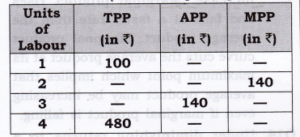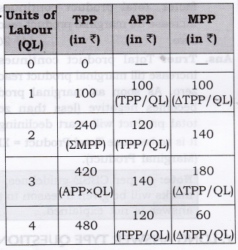# Chapter 5 – Production Questions and Answers: NCERT Solutions for Class 12 Micro Economics

Class 12 Introduction to Economics NCERT book solutions for Chapter 5 - Production Questions and Answers.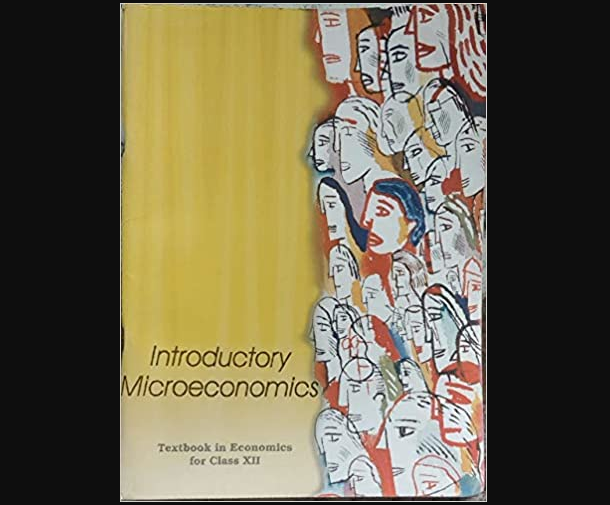## Answer: According to the Law of Variable Proportion when only one input is increased while all other inputs are kept constant, Marginal Product and Total Product behave in the following manner: 1. When Marginal product rises (till Point P1), Total product increases at an increasing rate (convex shape) (till point P). 2. When Marginal product falls and remains positive (Till point B1), total product increases at a diminishing rate (concave shape) (till point A), 3. When Marginal Product is zero (at point B1), Total Product is at its maximum and constant (At point B), 4. When Marginal product becomes negative (after point B1), total product falls (after point B).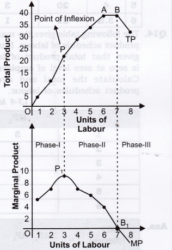## Question 9. The following table gives the total product schedule of labour. Find the corresponding average product and marginal product schedules of labour.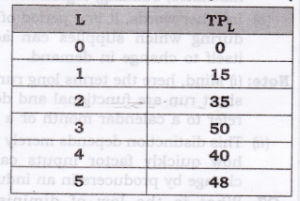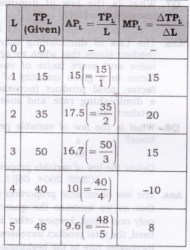## Question 10. The following table gives the average product schedule of labour. Find the total product and marginal product schedules. It is given that the total product is zero at zero level of labour employment.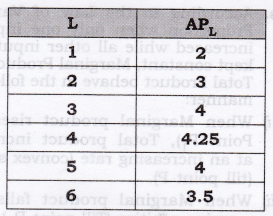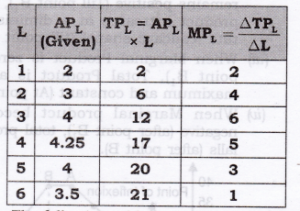## Question 11. The following table gives the marginal product schedule of labour. It is also given that total product of labour is zero at zero level of employment. Calculate the total and average product schedules of labour.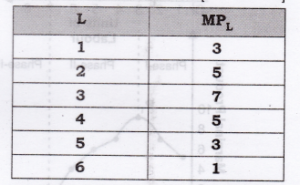## Question 1. Differentiate between Short Period and Long Period.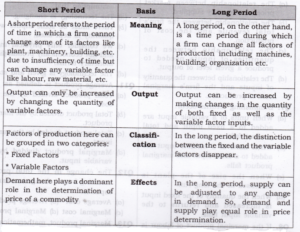## Question 2. Differentiate between Short Period production and Long Period production function.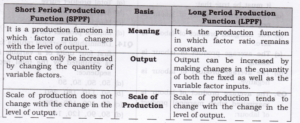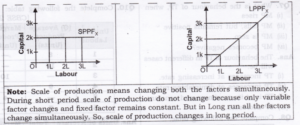## Question 3. Explain the relationship between Marginal product and Average Product. Or State the relation between Marginal product and average product. Use diagram.

### Answer: 1. Average Product increases as long as Marginal Product (MP) > Average Product (AP). Alternatively, when MP > AP, AP rises. 2. Average Product is maximum and constant when Average Product (AP) = Marginal Product (MP). Alternatively, when AP = MP, AP is maximum. 3. Average Product falls when Marginal Product < Average product. 4. Marginal Product can be zero and negative but Average product is never zero.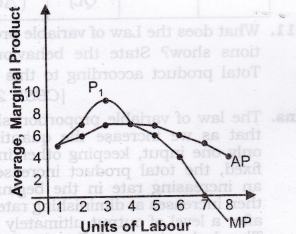## Question 8 . Complete the following table.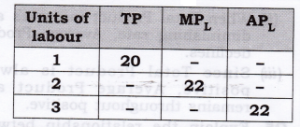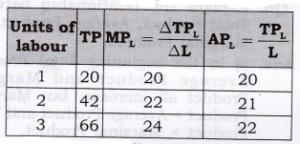##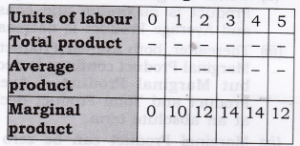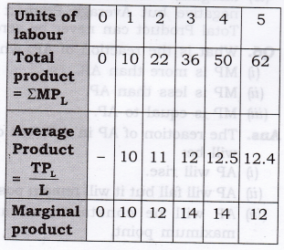## Question 11. What does the Law of variable proportions show? State the behaviour of Total product according to this Law.

### Answer: The law of variable proportion shows that as we increase the quantity of only one input, keeping other inputs fixed, the total product increases at an increasing rate in the beginning, then increases at diminishing rate and after a level of output ultimately falls. The behaviour of Total product according to this law is as under: 1. TP increases continuously from points O to A. 2. It increases at an increasing rate (convex shape) from O to P and at a diminishing rate (concave shape) from P to A. 3. TP is maximum at A and remains so up to point B. 4. After Point B, Total Product falls.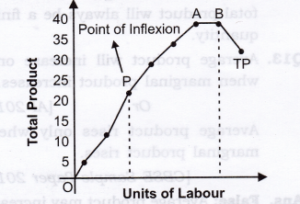## Question 12. Complete the following table: